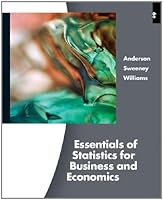# Essentials Of Stratistics For business & Economics, 6th Edition## Book Description

The Sixth Edition of ESSENTIALS OF FOR AND ECONOMICS is an introductory statistics book that emphasizes essential statistical concepts and their practical applications. The discussion and development of each technique are geared toward real-world applications, with the statistical results providing insights for decisions and solutions related to common problems. The easy-to-follow presentation style and proven problem-scenario approach clearly show how to apply statistical methods in practical situations. This brief introduction to statistics provides both a conceptual understanding of statistics and real-world applications of statistical methodology.

Chapter 1: Data and Statistics
Chapter 2: Descriptive Statistics: Tabular and Presentations
Chapter 3: Descriptive Statistics: Numerical Measures
Chapter 4: Introduction to Probability
Chapter 5: Discrete Probability Distributions
Chapter 6: Continuous Probability Distributions
Chapter 7: Sampling and Sampling Distributions
Chapter 8: Interval Estimation
Chapter 9: Hypothesis Tests
Chapter 10: Comparisons Involving Means, Experimental , and Analysis of Variance
Chapter 11: Comparisons Involving Proportions and a Test of Independence
Chapter 12: Simple Linear Regression
Chapter 13: Multiple Regression

Appendix A: References and Bibliography
Appendix B: Tables
Appendix C: Summation Notation
Appendix D: Self-Test Solutions and Answers to Even-Numbered Exercises
Appendix E: Using
Appendix F: Computing p-Values Using Minitab and Excel